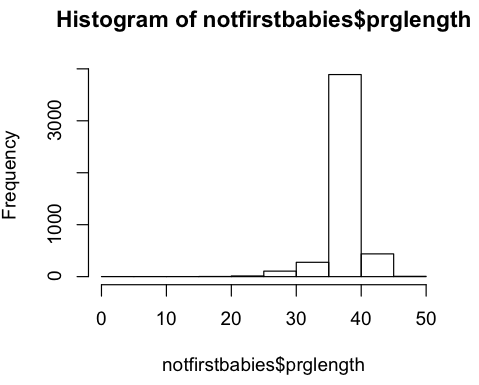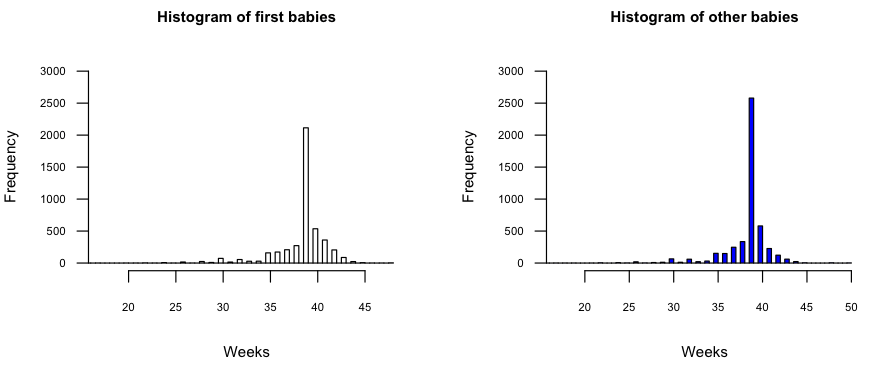# ThinkStats … in R :: Example/Chapter 2 :: Example 2.1-2.3

As promised, this post is a bit more graphical, but I feel the need to stress the importance of the first few points in chapter 2 of the book (i.e. the difference between mean and average and why variance is meaningful). These are fundamental concepts for future work.

The “pumpkin” example (2.1) gives us an opportunity to do some very basic R:

```1 2 3 4 pumpkins <- c(1,1,1,3,3,591) #build an array mean(pumpkins) #mean (average) var(pumpkins) #variance sd(pumpkins) #deviation```

(as you can see, I’m still trying to find the best way to embed R source code)

We move from pumpkins to babies for Example 2.2 (you’ll need the whole bit of source from previous examples (that includes all the solutions in this example) to make the rest of the code snippets work). Here, we can quickly compute and compare the standard deviations (with difference) and the means (with difference) to help us analyze the statistical significane questions in the chapter:

```1 2 3 4 5 6 7 sd(firstbabies\$prglength) sd(notfirstbabies\$prglength) sd(firstbabies\$prglength) - sd(notfirstbabies\$prglength)   mean(firstbabies\$prglength) mean(notfirstbabies\$prglength) mean(firstbabies\$prglength) - mean(notfirstbabies\$prglength)```

You’ll see the power of R’s `hist` function in a moment, but you should be a bit surprised when you see the output if you enter to solve Example 2.3:

```1 mode(firstbabies\$prglength)```

That’s right, R does not have a built-in `mode` function. It’s pretty straightforward to compute, tho:

```1 names(sort(-table(firstbabies\$prglength)))```

(notice how “straightforward” != “simple”)

We have to use the `table` function to generate a table of value frequencies. It’s a two-dimensional structure with the actual value associated with the frequency represented as a string indexed at the same position. Using “`-`” inverts all the values (but keeps the two-dimensional indexing consistent) and `sort` orders the structure so we can use index “``” to get to the value we’re looking for. By using the `names` function, we get the string representing the value at the highest frequency. You can see this iteratively by breaking out the code:

```1 2 3 4 5 6 table(firstbabies\$prglength) str(table(firstbabies\$prglength)) sort(table(firstbabies\$prglength)) sort(table(firstbabies\$prglength)) #without the "-" sort(-table(firstbabies\$prglength)) names(sort(-table(firstbabies\$prglength)))```

There are a plethora of other ways to compute the `mode`, but this one seems to work well for my needs.

### Pictures Or It Didn’t Happen

I did debate putting the rest of this post into a separate example, but if you’ve stuck through this far, you deserve some stats candy. It’s actually pretty tricky to do what the book does here:So, we’ll start off with simple histogram plots of each set being compared:

```1 hist(firstbabies\$prglength)``````1 hist(notfirstbabies\$prglength)```I separated those out since `hist` by default displays the histogram and if you just paste the lines consecutively, you’ll only see the last histogram. What does display is, well, ugly and charts should be beautiful. It will take a bit to explain the details (in another post) but this should get you started:

```1 2 3 4 par(mfrow=c(1,2))par(mfrow=c(1,2)) hist(firstbabies\$prglength, cex.lab=0.8, cex.axis=0.6, cex.main=0.8, las=1, col="white", ylim=c(0,3000),xlim=c(17,max(firstbabies\$prglength)), breaks="Scott", main="Histogram of first babies", xlab="Weeks") hist(notfirstbabies\$prglength, cex.lab=0.8, cex.axis=0.6, cex.main=0.8, las=1, col="blue", ylim=c(0,3000),xlim=c(17,max(notfirstbabies\$prglength)), breaks="Scott", main="Histogram of other babies", xlab="Weeks") par(mfrow=c(1,1))```

In the above code, we’re telling R to setup a canvas that will have one row and two plot areas. This makes it very easy to have many graphs on one canvas.

Next, the first `hist` sets up up some label proportions (the `cex` parameters), tells R to make Y labels horizontal (`las=1`), makes the bars white, sets up sane values for the X & Y axes, instructs R to use the “Scott” algorithm for calculating sane bins (we’ll cover this in more details next post) and sets up sane titles and X axis labels. Finally, we reset the canvas for the next plot.

There’s quite a bit to play with there and you can use the “`help()`” command to get information on the `hist` function and `plot` function. You can setup your own bin size by substituting an array for “Scott”. If you have specific questions, shoot a note in the comments, but I’ll explain more about what’s going on in the next post as we add in probability histograms and start looking at the data in more detail.Click for larger image